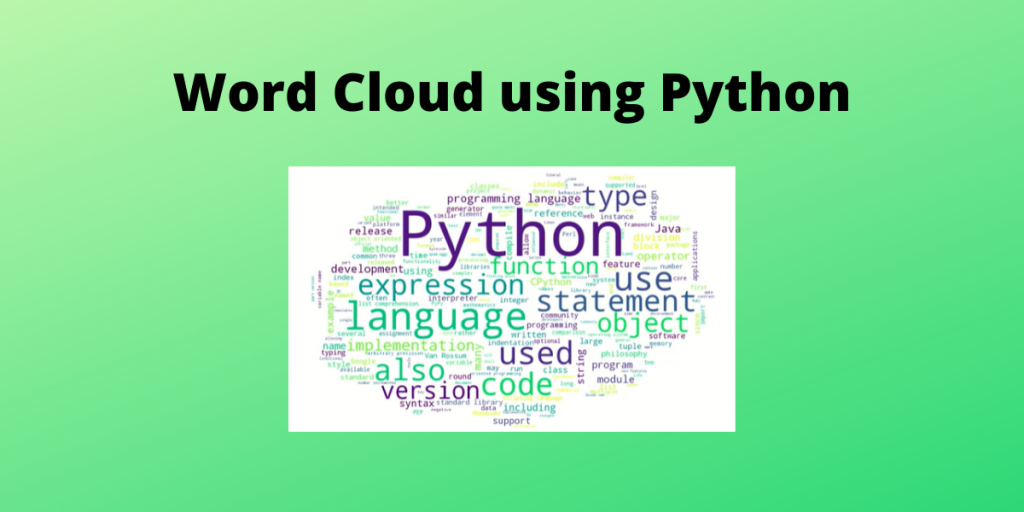# Word Cloud using PythonWelcome to this tutorial on word cloud using Python. The word cloud technique has been a trending technique of data visualization, especially where textual data is present.

Hence, we can say that Word Cloud has been one of the prominent techniques for data visualization using Natural Language Processing (NLP).

## What is a Word Cloud?

We extract the most frequently used words in the article and then based on the number of times a word is used.

Greater the usage, greater the size of the word in the word cloud.

## How to Create a Word Cloud using Python?

So, lets begin with creating our own word cloud using Python.

### 1. Install the wordcloud and Wikipedia libraries

To create a word cloud, we need to have python 3.x on our machines and also wordcloud installed. To install wordcloud, you can use the pip command:

```sudo pip install wordcloud
```

For this example, I will be using a webpage from Wikipedia namely – Python (programming language). To use Wikipedia contents, we need to install the wikipedia dependencies.

```sudo pip install wikipedia
```

### 2. Search Wikipedia based on a query

First, we will import the `wikipedia` library using the code snippet below:

```import wikipedia
```

We will use the `search` function and only take the first element out of it, this is why we use . This will be the title of our page.

```def get_wiki(query):
title = wikipedia.search(query)

page = wikipedia.page(title)
return page.content
```

After extracting the `title`, we use the `page()` and retrieve the contents of the page. After this we return only the `content` of the page using `page.content`.

If you run the above code on the console, you will get all the raw data from the site on the console. But our task does not end here, we need to make a word cloud.

### 3. Create cloud mask and set stop words

To begin with we will import the `wordcloud` library and import specific packages such as `WordCloud` and `STOPWORDS`.

We import the `STOPWORDS` because we want to remove basic articles such as a,an,the and other common words used in the English Language.

```from wordcloud import WordCloud, STOPWORDS
```

We will use the `mask`. This a rough diagram named as ‘cloud.png’ in the current working directory denoted by `currdir`. We will open this image and store it in a numpy array.

Our next task is to define a set of stopwords and hence we use `set(STOPWORDS)`.

We create the word cloud using a Python object using the `WordCloud`(). We will pass parameters such as `background_color`, `max_words` (here we choose our word limit as 200), `mask` and `stopwords`.

We will then use the `wc.generate()` and pass the raw text as a parameter.

We can also save the word cloud generated into a file and we will name it as `output.png`.

```def create_wordcloud(text):

stopwords = set(STOPWORDS)

# create wordcloud object
wc = WordCloud(background_color="white",
max_words=200,
stopwords=stopwords)

wc.generate(text)

# save wordcloud
wc.to_file(path.join(currdir, "output.png"))

```

Running these 2 functions may take upto 30-40 seconds the first time, and may reduce over further runs. The complete code and output image is as shown below in the next section.

## Complete Implementation of Word Cloud using Python

```import sys
from os import path
import numpy as np
from PIL import Image
import wikipedia
from wordcloud import WordCloud, STOPWORDS

currdir = path.dirname(__file__)

def get_wiki(query):
title = wikipedia.search(query)
page = wikipedia.page(title)
return page.content

def create_wordcloud(text):

stopwords = set(STOPWORDS)

wc = WordCloud(background_color="white",
max_words=200,
stopwords=stopwords)

wc.generate(text)
wc.to_file(path.join(currdir, "output.png"))

if __name__ == "__main__":
query = sys.argv
text = get_wiki(query)

create_wordcloud(text)
```

Output: